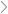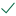Questions & AnswersCivil EngineeringQuestion 3 The following information on the upper floor slabs are...

QuestionAnswered step-by-step

# Question 3 The following information on the upper floor slabs are...

Question 3
The following information on the upper floor slabs are obtained from the cost analysis
of a past project:
 4 storey building
 200mm concrete upper floor slabs
 GFA is 3200m2 (4 floors, each of 800m2)
 Element Rate is \$158/m2
 Cost Index is 300
The following information are for the upper floors of a proposed building:
 5 storey building
 250mm concrete upper floor slabs
 GFA = 3000 m2 (5 floors, each of 600m2)
 Current Cost Index is 320
(a) Appraise the previous project information and determine the:
(i) Elemental cost of the upper floors for the proposed building.
(5 marks)
(ii) Elemental unit rate of the upper floors for the proposed building.
(5 marks)
(iii) Element rate of the upper floors for the proposed building.
(5 marks)
(b) Explain the differences between Elemental Cost; Element Unit Rate and Element
Rate.
(5 marks)Solved by verified expert10,000 step-by-step explanations